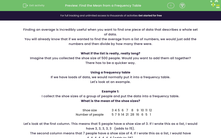# Find the Mean from a Frequency Table

In this worksheet, students will find the mean from a frequency table.Key stage:  KS 4

Year:  GCSE

GCSE Subjects:   Maths

GCSE Boards:   AQA, Eduqas, Pearson Edexcel, OCR,

Curriculum topic:   Statistics

Curriculum subtopic:   Statistics Analysing Data

Difficulty level:#### Worksheet Overview

Finding an average is incredibly useful when you want to find one piece of data that describes a whole set of data.

You will already know that if we wanted to find the average from a list of numbers, we would just add the numbers and then divide by how many there were.

What if the list is really, really long?

Imagine that you collected the shoe size of 500 people. Would you want to add them all together?

There has to be a quicker way.

Using a frequency table

If we have loads of data, we would normally put it into a frequency table.

Let's look at an example.

Example 1:

I collect the shoe sizes of a group of people and put the data into a frequency table.

What is the mean of the shoe sizes?

 Shoe size 3 4 5 6 7 8 9 10 11 12 Number of people 5 7 9 14 21 28 16 6 5 1

Let's look at the first column. This means that 5 people have a shoe size of 3. If I wrote this as a list, I would have 3, 3, 3, 3, 3   (adds to 15).

The second column means that 7 people have a shoe size of 4. If I wrote this as a list, I would have

4, 4, 4, 4, 4, 4, 4 (adds to 28).

To find the total, all I need to do to start with is to multiply the two numbers together!

For example: 5 x 3 = 15 and 7 x 4 = 28.

If I did this for all of them, I would get:

 Shoe size 3 4 5 6 7 8 9 10 11 12 Number of people 5 7 9 14 21 28 16 6 5 1 15 28 45 84 147 224 144 60 55 12

Once we have this, we can work out what all the shoe sizes added together would be by adding the numbers in red. (814 )

We now have one of the bits of information we need to find the mean (what they all add up to).

All we need now is to find out how many people were asked. We can get this by adding up all the numbers in the number of people row. (112)

To find the mean, all we now have to do is to divide one of these numbers by the other: 814 ÷ 112 to get an average of 7.3.

A couple of points

Does it matter that the answer is a decimal because you can't have a shoe size of 7.3.

No, it doesn't matter because it's not a real shoe - it just represents all the others, so decimals are fine.

How do I know which way round to divide?

If you can't remember, try them both ways round. You'll get two answers: 7.3 and 0.14.

Remember that the average must be in the middle(ish) of the data, so it must be 7.3.

To summarise:

Step 1: Multiply the two columns together.

Step 2: Add all these new numbers up.

Step 3: Add up all the frequencies.

Step 4: Divide to find the mean.

Let's try some questions now.

### What is EdPlace?

We're your National Curriculum aligned online education content provider helping each child succeed in English, maths and science from year 1 to GCSE. With an EdPlace account you’ll be able to track and measure progress, helping each child achieve their best. We build confidence and attainment by personalising each child’s learning at a level that suits them.

Get started